## Cipher## Mar 15, 2017

### CNN - Maria Sharapova suspension, winning Wimbledon 2017, incredible coding

CNN's story -

A few months ago I saw a story on Sharapova and had some pretty interesting finds in relation to her "drug ban" so I decided to wait for the next story which is the one coming today on March 15th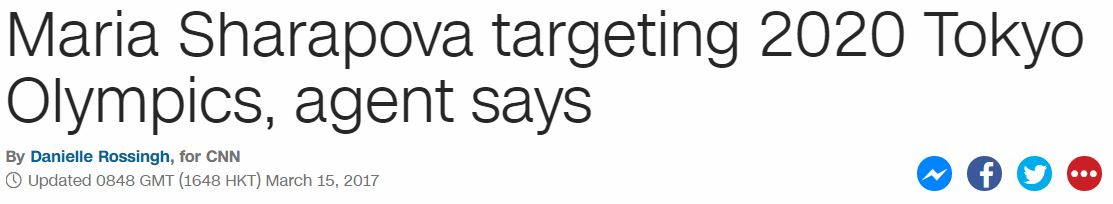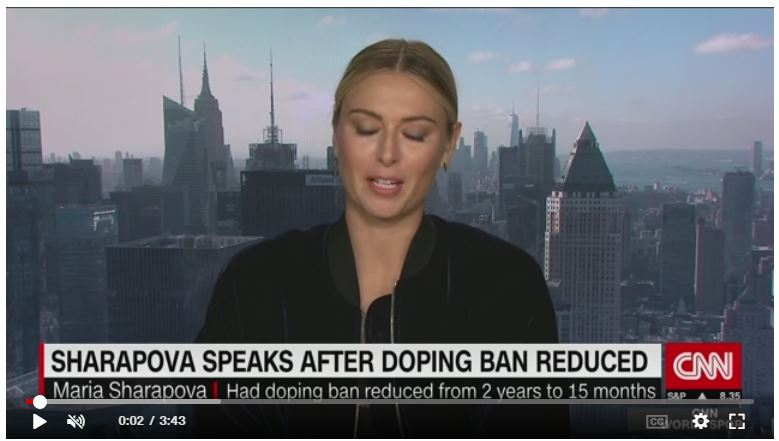Note the length of the video - 3:43 (7x7x7) , she failed a drug test on 26th of January - 3:43 is 223 seconds (The Synagogue of Satan = 223, the number of Skull and Bones is 322)
Twenty sixth of January = 100+900+5+40+100+400 + 90+9+300+100+8 + 50+6 + 600+1+40+200+1+80+400 = 3430 (Jewish)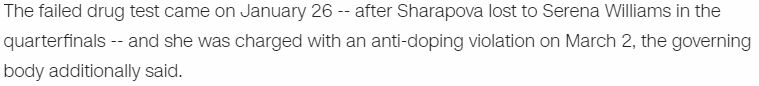1/26/16 = 1+26+16 = 43 (The news of the failed test came 42 days later on 3/7/2016 -  "Maria" = 13+1+18+9+1 = 42 (Ordinal), "Sharapova" = 8+19+26+9+26+11+12+5+26 = 142 (Reverse Ordinal)"Maria Sharapova" = 1+1+5+5+1 + 6+6+1+5+1+3+2+5+1 = 43 (Septenary)
She tested positive for "Meldonium" = 4+5+3+4+6+5+9+3+4 = 43 (Reduced)
The mainstream media started covering story about failed test on March 7th, which was also 43 days before her next birthday
"March" = 13+1+18+3+8 = 43 (Ordinal)She's born on 19th of April
Nineteenth of April = 14+9+14+5+20+5+5+14+20+8 + 15+6 + 1+16+18+9+12 = 191 (Ordinal)Her full name is "Maria Yuryevna Sharapova" = 4+1+9+9+1 + 7+3+9+7+5+4+5+1 + 10+8+1+9+1+7+6+4+1 = 112 (S Exception)"Sharapova eligible to return on April 26" = 45+8+1+18+1+16+15+22+1 + 5+12+9+7+9+2+12+5+20+15+18+5+20+21+18+14+15+14+27+16+18+9+12 = 430 (Francis Bacon)
"Twenty sixth of April" = 2+5+5+5+2+7 + 1+9+6+2+8 + 6+6 + 1+7+9+9+3 = 93 (Reduced)
"Maria" = 14+26+9+18+26 = 93 (Reverse Ordinal)
From the release of this story there are 43 days to April 26th
April = 8+2+9+9+6 = 34 (Reverse Reduced)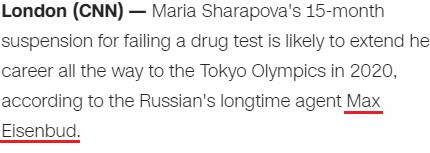Max = 14+26+3 = 43 (Reverse Ordinal)
Eisenbud = 5+9+10+5+5+2+3+4 = 43 (S Exception)
Maria Sharapova = 13+1+18+9+1 + 19+8+1+18+1+16+15+22+1 = 143 (Ordinal)
On the wikipedia we find that she has lost 43 games in Grand Slam TournamentsHer name and birthday have the same gematria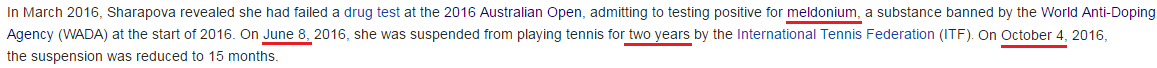"Two year ban" = 2+5+6 + 7+5+1+9 + 2+1+5 = 43 (Reduced)
She was suspended on 6/8 and then on 10/4 they decided to reduce the ban to 15 months
6+8 = 14 (6+8+20+16 = 50)
10+4 = 14 , both of these dates have date numerology of 50 (10+4+20+16) - "Circle" = 3+9+18+3+12+5 = 50 (Ordinal), America = 1+13+5+18+9+3+1 = 50 (Ordinal)
43 is the 14th prime number
Maria made her professional tennis debut in 2001 at the age of 14
In 2017 it's going to be her 14th time on Wimbledon (43)

From 6/8/2016 to 10/4/2016 is 119 days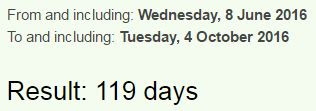She was suspended 135 days after failing a drug test (135+531 = 666) - Every angle in an octagon has 135° - one hundred thirty five = 60+50+5 + 8+300+50+4+90+5+4 + 200+8+9+90+200+700 + 6+9+400+5 = 2203 (English)From March 7th release of failed test to her suspension is 93 daysThere's definitely many connections with Saturnian numbers here
Saturn = 19+1+20+21+18+14 = 93 (Ordinal)
Saturn = 45+1+20+21+18+14 = 119 (Francis Bacon)
Star of David = 19+20+1+18 + 15+6 + 4+1+22+9+4 = 119 (Ordinal)
All Seeing eye = 1+12+12 + 19+5+5+9+14+7 + 5+25+5 = 119 (Ordinal)...

Twenty twenty Tokyo Olympics = 7+4+4+4+7+2 + 7+4+4+4+7+2 + 7+3+7+2+3 + 3+6+2+5+2+9+6+8 = 119 (Reverse Reduced)

The last tournament she played was "Australian Open" = 1+3+10+2+9+1+3+9+1+5 + 6+7+5+5 = 67 (S Exception)
Information about her failed test was released on 67th day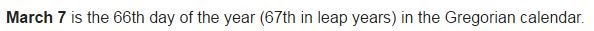sixty seventh day = 1+9+6+2+7 + 1+5+4+5+5+2+8 + 4+1+7 = 67 (Reduced)
"Seventh of March" = 1+5+4+5+5+2+8 + 6+6 + 4+1+9+3+8 = 67 (Reduced)
Her ban was shortened on "October Fourth" = 6+3+2+6+2+5+9 + 6+6+3+9+2+8 = 67 (Reduced)Stuttgart, Germany = 1+2+3+2+2+7+1+9+2 + 7+5+9+4+1+5+7 = 67 (Reduced)
April 26 is a date with 67 numerology (4+26+20+17)

From March 7th to October 4th is 211 days (47th prime number)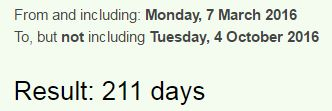33 and 47 are the numbers that often go together and their primes have a relationship through octal system, also there's a lot of 112 coding with this story, the reflection of 211
"Wimbledon" = 4+9+5+7+6+4+5+3+4 = 47 (Reverse Reduced)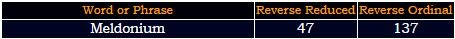This year Wimbledon is starting on 7/3 with a date numerology of 47 (7+3+20+17) and it ends on a date with 33 numerology 7/16/2017 (7+16+2+0+1+7)

From the suspension (6/8/2016) to her game in April is 322 days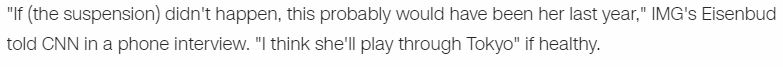Notice how in the next part of this story they are saying how she is healthy and recovered from the injuries and now her career is going to be extended because of the rest she got from the suspension. I think the entire reason they put Meldonium on the list at the start of 2016 is to have her suspended in order to have her play for a few more years... and based on this coding I would say she is going to win both Wimbledon 2017 and Tokyo Olympics. In regards to Meldonium, some experts say that there is no evidence that indicates it is a performance enhancer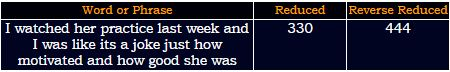Wimbledon = 5+9+4+2+3+5+4+6+5 = 43 (Reduced)
Wimbledon is starting on July 3rd and it ends on the 16th (Sixteen = 33 - Ordinal)
Sixteenth = 1+9+6+2+5+5+5+2+8 = 43 (Reduced)
Her birthday this year is landing on a date with 33 numerology (4+19+2+0+1+7) and the Wimbledon is ending on a date that has exactly the same date numerology as her birthdayJuly Sixteenth = 1+3+3+7 + 10+9+6+2+5+5+5+2+8 = 66 (S Exception)66 decimal places of Pi sum to 322
156th Fibonacci number has 33 digits - 178890334785183168257455287891792

She failed a drug test when she was "Twenty eight" = 20+23+5+14+20+25 + 5+9+7+8+20 = 156 (Ordinal)
Also she was banned by "International Tennis Federation"Thirty three = 7+19+18+9+7+2 + 7+19+9+22+22 = 141 (Reverse Ordinal)
Her first game after suspension is at Porsche Tennis Grand Prix tournament in Stuttgart
Porsche Tennis Grand Prix = 7+6+9+10+3+8+5 + 2+5+5+5+9+10 + 7+9+1+5+4 + 7+9+9+6 = 141 (S Exception)

The tournament is starting on 4/22 and it ends on 4/30April 22nd has date numerology of 43 like connecting to everything mentioned above
4/22/17 - 4+22+17 = 43 , she failed a drug test on 1/26/16 = 1+26+16 = 43
Tournament ends on 4/30 = 34
If she wins (and she probably will) it will be her fourth title on Stuttgart tournament
"fourth title" = 21+12+6+9+7+19 + 7+18+7+15+22 = 143 (Reverse Ordinal)
"Maria Sharapova" = 13+1+18+9+1 + 19+8+1+18+1+16+15+22+1 = 143 (Ordinal)

Porsche Tennis Grand Prix = 11+12+9+8+24+19+22 + 7+22+13+13+18+8 + 20+9+26+13+23 + 11+9+18+3 = 318 (Reverse Ordinal)
7/22 = 0.318

There are 2 months and 27 days from her birthday to the end of Wimbledon 2017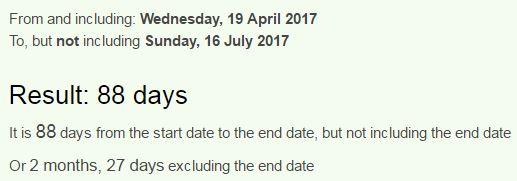22/7 = 3.14
From 3/15/2017 to her birthday is 1 month and 12 days a lot like 112
"Circle" = 24+18+9+24+15+22 = 112 (Reverse Ordinal)
"Olympics" = 15+12+25+13+16+9+3+19 = 112 (Ordinal)
"Tokyo" = 46+15+11+25+15 = 112 (Francis Bacon)
The Mainstream media started covering her story 112 days before the start of Wimbledon 2016It's very interesting how they started talking about her on 3/7 and Wimbledon in 2017 is coming on 7/3...
This would be her "sixth championship" = 112 (S Exception) title
"Maria Yuryevna Sharapova" = 4+1+9+9+1 + 7+3+9+7+5+4+5+1 + 10+8+1+9+1+7+6+4+1 = 112 (S Exception)

Think about how Maria Sharapova = 143 (Ordinal), missed Rio Olympics = 143 (Reverse Ordinal) last year
The rest of 2017 might be the best year of her career, her alignment with the tournaments is completely ridiculous

#### 1 comment:

1.